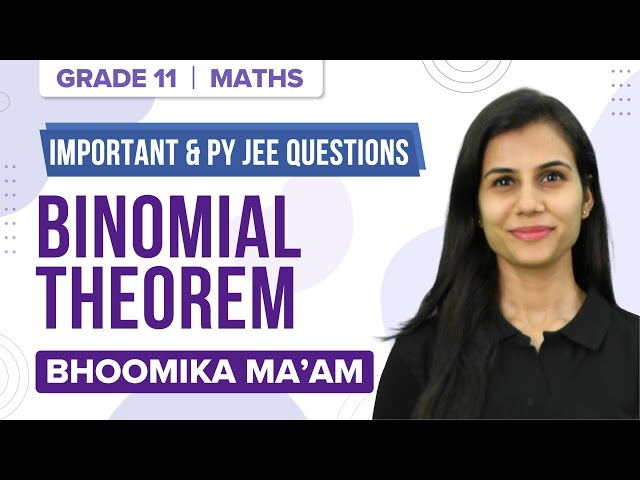Win up to 100% scholarship on Aakash BYJU'S JEE/NEET courses with ABNAT Win up to 100% scholarship on Aakash BYJU'S JEE/NEET courses with ABNAT

# JEE Advanced Maths Binomial Theorem Previous Year Questions with Solutions

Students can find the JEE Advanced Maths binomial theorem, previous years’ questions and solutions on this page. Expansion of binomial, general term, coefficient of any power of x, properties of binomial coefficients, number of terms in an expansion, etc., are the important topics in binomial theorem. Practising the JEE Advanced Maths binomial theorem questions and solutions will help students become familiar with the type of questions asked from this topic.

Question 1: Coefficient of x11 in the expansion (1 + x2)4 (1 + x3)7 (1 + x4)12 is

(a) 1051

(b) 1106

(c) 1113

(d) 1120

Solution:

(1 + x2)4 (1 + x3)7 (1 + x4)12

=> coefficient of xa × coefficient of xb × coefficient of xc

So that a+b+c = 1

Here a = 2m, b = 3n, c = 4p

So 2m + 3n + 4p = 11

Possibilities are m = 0, n = 1, p = 2

m = 1, n = 3, p = 0

m = 2, n = 1, p = 1

m = 4, n = 1, p = 0

Required coefficient = 4C0×7C1×12C2 + 4C1×7C3×12C0 + 4C2×7C1×12C1 + 4C4×7C1×12C0

= 462 + 140 + 504 + 7

= 1113

Hence option c is the answer.

Question 2: The coefficients of three consecutive terms of (1+x)n+5 are in the ratio 5: 10: 14. Then n =

Solution:

Tr+1 = n+5Crxr

Given n+5Cr-1 : n+5Cr : n+5Cr+1 = 5: 10: 14

n+5Cr/ n+5Cr-1 = 10/5

=> (n+5-r+1)/r = 2

=> (n+6-r)/r = 2

=> n+6-r = 2r

n = 3r-6 ..(i)

n+5Cr+1/ n+5Cr = 14/10

=> ((n+5)-(r+1)+1)/(r+1) = 7/5

=> (n+5-r)/(r+1) = 7/5

=> 5n+25-5r = 7r + 7

=> 5n – 12r + 18 = 0 ..(ii)

Put (i) in (ii)

5(3r-6) – 12r + 18 = 0

=> 15r – 30 – 12r + 18 = 0

=> 3r – 12 = 0

=> r = 4

So n = 3r-6

= 12-6

= 6

Hence the value of n is 6.

Question 3: The coefficient of x9 in the expansion (1+x)(1+x2)(1+x3) ..(1+x100) is

Solution:

Given expansion (1+x)(1+x2)(1+x3) ..(1+x100)

To get power 9, the possibilities are

(0, 9), (1, 8), (2, 7), (3, 6), (4, 5) = 5 ways

Also (1, 2, 6), (1, 3, 5), (2, 3, 5) = 3 ways

So the coefficient of x9 in the given expression is 8.

Question 4: If {p} denotes the fractional part of the number p, then {3200/8}, is equal to

(a) 5/8

(b) ⅞

(c) ⅜

(d) 1/8

Solution:

3200/8 = (⅛)9100

= ⅛ (1 + 8)100

= ⅛ (1 + 100C1.8 + 100C2.82 + …+100C100.8100)

= 1/8 + integer

So {1/8 + integer} = 1/8

Hence option d is the answer.

Question 5: The coefficient of x7 in the expression (1 + x)10 + x(1 + x)9 + x2(1 + x)8 + ⋯ + x10 is :

(a) 420

(b) 330

(c) 210

(d) 120

Solution:

Let S= (1+x)10+ x(1+x)9+ x2(1+x)8+⋯ +x10

Applying sum of terms of G.P

S = (1+x)10(1 – (x/(1+x))11 )/(1 – x/(1+x))

= (1 + x)11 – x11

Coefficient of x7 is 11C7 = 11!/7! 4!

= 330

Hence option b is the answer.

Question 6: The coefficient of x4 in the expansion of (1+x+x2)10 is

Solution:

General term of the given expression is given by (10!/p!q!r!)xq+2r

Here, q+2r = 4

When p = 6, q = 4, r = 0, coefficient = 10!/(6!×4!) = 210

When p = 7, q = 2, r = 1, coefficient = 10!/(7!×2!×1!) = 360

When p = 8, q = 0, r = 2, coefficient = 10!/(8!×2!) = 45

Sum = 615

Question 7: If Cr = 25Cr and C0+5⋅C1+9⋅C2 +⋯+101. C25 =225.k then k is equal to

Solution:

S = 25C0 + 5 25C1 + 9 25C2 + ….+97 25C24 +101 25C25 = 225k ..(i)

Reverse and apply property nCr = nCn-r in all coefficients

S = 101 25C0 + 97 25C1+…+5 25C24+25C25 …(ii)

2S = 102 [25C0+25C1+…+25C5]

S = 51×225

k = 51

Question 8: If the fractional part of the number 2403/15 is k/15, then k is equal to:

(a) 4

(b) 8

(c) 6

(d) 14

Solution:

2403 = 2400 × 23

= (24)100×8

= 8×16100

= 8(1 + 15)100

When 2403 is divided by 15

=> (8/15)(1+15x) = 8x + 8/15

So fractional part of the number is 8/15.

So k = 8

Hence option b is the answer.

Question 9: The number of terms in the expansion of the (1+x)101(1+x2 -x)100 in powers of x is

(a) 302

(b) 301

(c) 202

(d) 101

Solution:

(1+x)101(1+x2 -x)100 = (1+x)(1+x)100(1-x+x2)100

= (1+x)[(1+x)(1-x+x2)]100

= (1+x)(1+x3)100

(1+x3)100 will have 100+1 = 101 terms

So (1+x)(1+x3)100 will have 2×101 = 202 terms

Hence option c is the answer.

Question 10: The sum of the rational terms in the binomial expansion of (21/2 + 31/5)10 is

(a) 25

(b) 32

(c) 9

(d) 41

Solution:

(21/2 + 31/5)10 = 10C0(21/2)10 + 10C1(21/2)9(31/5) + … + 10C10(31/5)10

Two rational terms are there. First and last term.

Sum of two rational terms = 25 + 32

= 32 + 9

= 41

Hence option d is the answer.

Recommended video